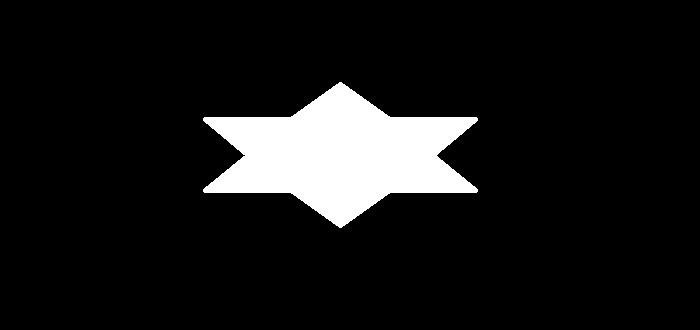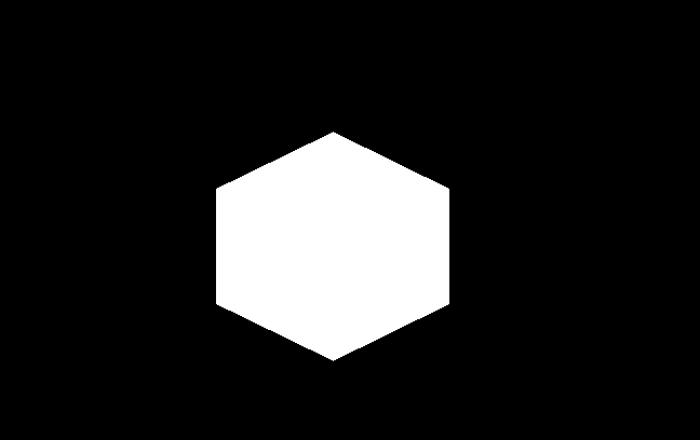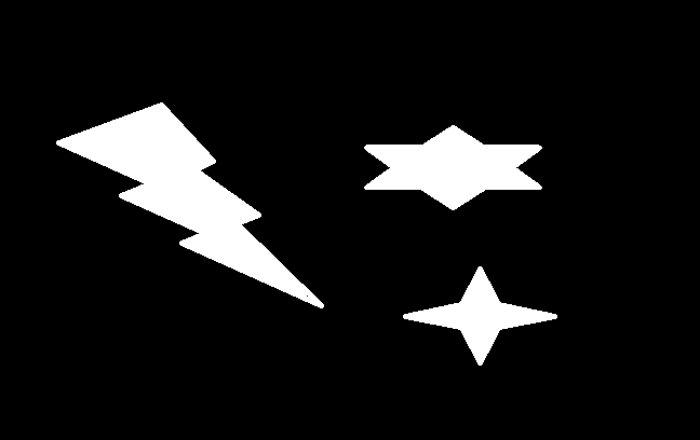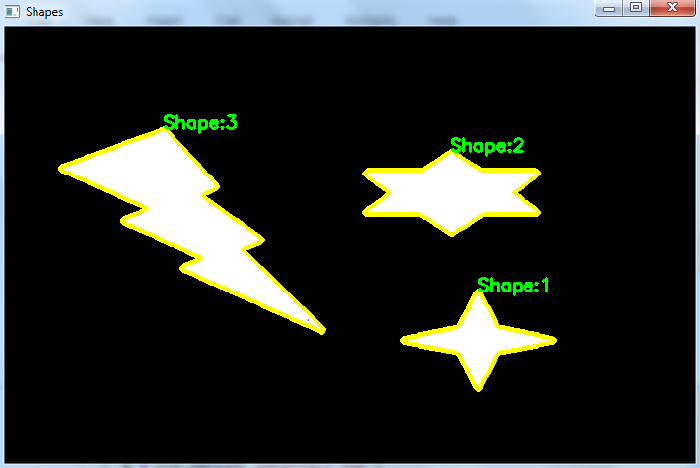# How to match image shapes in OpenCV Python?

We use cv2.matchShapes() function to match two image shapes. This function returns a metric showing the similarity between the image shapes. This function uses Hu-Moments to calculate the metric value. Lower the metric value, higher the similarity between the image shapes.

In the following examples, we will match the shapes from different images and also shapes from a single image.

## Syntax

We use the following syntax to match two image shapes −

ret = cv2.matchShapes(cnt1,cnt1,1,0.0)


Where,

• cnt1 − The contour points of the first image shape.

• cnt2 − The contour points of the second image shape

## Steps

You can use the following steps to match two image shapes −

Import the required library. In all the following Python examples, the required Python library is OpenCV. Make sure you have already installed it.

import cv2


img1 = cv2.imread('star.png',0)


Apply thresholding on the grayscale images to create binary images.

ret,thresh1 = cv2.threshold(img1,150,255,0)
ret,thresh2 = cv2.threshold(img1,150,255,0)


Find the contours of the shapes in the binary images using cv2.findContours() function.

contours1, _ = cv2.findContours(thresh1, cv2.RETR_TREE, cv2.CHAIN_APPROX_SIMPLE)
contours2, _ = cv2.findContours(thresh2, cv2.RETR_TREE, cv2.CHAIN_APPROX_SIMPLE)


Select the particular contour from each image and apply the shape match function cv2.matchShapes() passing the selected contours.

cnt1=contours1
cnt2=contours2
ret12 = cv2.matchShapes(cnt1, cnt2, 1, 0.0)


Print the result value, the image shape matching metric. The lower the value, the better matching it is.

print("Matching Image 1 with Image 2:", ret12)


Let's have a look at some examples for a better understanding.

## Example 1

In this program, we match two image shapes. Each image contains a single shape. We also match the shape with itself from each image.

# import required libraries
import cv2

# Read two images as grayscale images

# Apply thresholding on the images to convert to binary images
ret, thresh1 = cv2.threshold(img1, 127, 255,0)
ret, thresh2 = cv2.threshold(img2, 127, 255,0)

# find the contours in the binary image
contours1,hierarchy = cv2.findContours(thresh1,cv2.RETR_TREE,cv2.CHAIN_APPROX_SIMPLE)
print("Number of Shapes detected in Image 1:",len(contours))
cnt1 = contours1

contours2,hierarchy = cv2.findContours(thresh2,cv2.RETR_TREE,cv2.CHAIN_APPROX_SIMPLE)
print("Number of Shapes detected in Image 2:",len(contours))
cnt2 = contours2

# Compute the match scores
ret11 = cv2.matchShapes(cnt1,cnt1,1,0.0)
ret22 = cv2.matchShapes(cnt2,cnt2,1,0.0)
ret12 = cv2.matchShapes(cnt1,cnt2,1,0.0)

# print the matching scores
print("Matching Image 1 with itself:", ret11)
print("Matching Image 2 with itself:", ret22)
print("Matching Image 1 with Image 2:", ret12)


Consider the below images as the input images mentioned as 'star.png' and 'pentagon.png' in the above program.## Output

On execution, the above code will produce the following output

Number of Shapes detected in Image 1: 1
Number of Shapes detected in Image 2: 1
Matching Image 1 with itself: 0.0
Matching Image 2 with itself: 0.0
Matching Image 1 with Image 2: 0.6015851094057714


## Example 2

In this program, we match the shapes in the image. We detect three shapes in the image.

import cv2
import numpy as np
gray = cv2.cvtColor(img, cv2.COLOR_BGR2GRAY)

ret,thresh = cv2.threshold(gray,100,255,0)
contours,hierarchy = cv2.findContours(thresh, cv2.RETR_TREE,cv2.CHAIN_APPROX_SIMPLE)
print("Number of Shapes detected:",len(contours))

# draw contour and shape number
for i, cnt in enumerate(contours):
M = cv2.moments(cnt)
x1, y1 = cnt[0,0]
img1 = cv2.drawContours(img, [cnt], -1, (0,255,255), 3)
cv2.putText(img1, f'Shape:{i+1}', (x1, y1), cv2.FONT_HERSHEY_SIMPLEX, 0.6, (0, 255, 0), 2)

cnt1 = contours
cnt2 = contours
cnt3= contours

ret11 = cv2.matchShapes(cnt1,cnt1,1,0.0)
ret12 = cv2.matchShapes(cnt1,cnt2,1,0.0)
ret23 = cv2.matchShapes(cnt2,cnt3,1,0.0)
ret31 = cv2.matchShapes(cnt3,cnt1,1,0.0)

print("Matching Shape 1 with itself:", ret11)
print("Matching Shape 1 with Shape 2:", ret12)
print("Matching Shape 2 with Shape 3:", ret23)
print("Matching Shape 3 with Shape 1:", ret31)

cv2.imshow("Shapes", img)
cv2.waitKey(0)
cv2.destroyAllWindows()


We will use the following image and the Input File in this program −## Output

On execution, the above code will produce the following output

Number of Shapes detected: 3
Matching Shape 1 with itself: 0.0
Matching Shape 1 with Shape 2: 0.15261042892128207
Matching Shape 2 with Shape 3: 0.9192709496955178
Matching Shape 3 with Shape 1: 0.7521097407160106


And we get the following window, showing the output −On the basis of the above result, we conclude that the Shape 1 is more similar to Shape 2 than Shape 3.

Updated on: 28-Sep-2022

4K+ Views